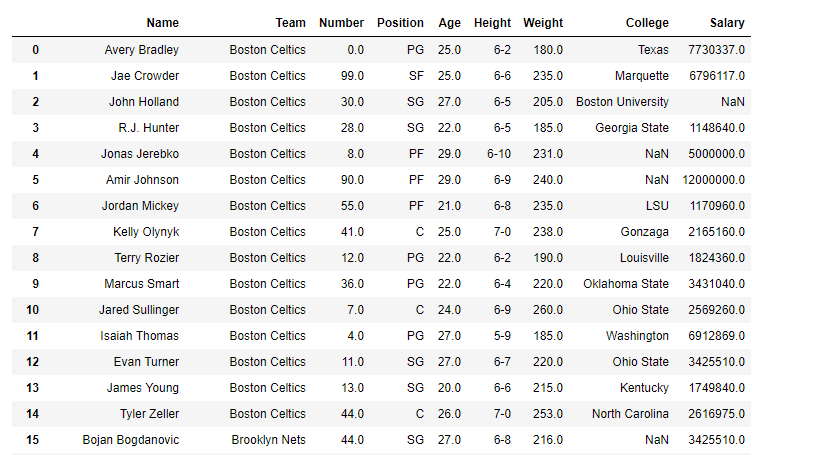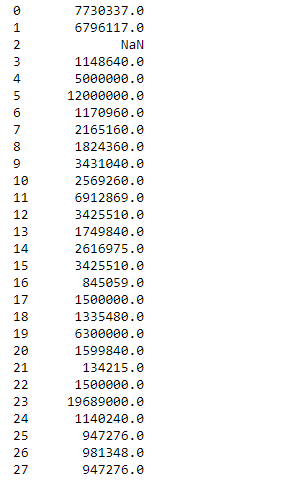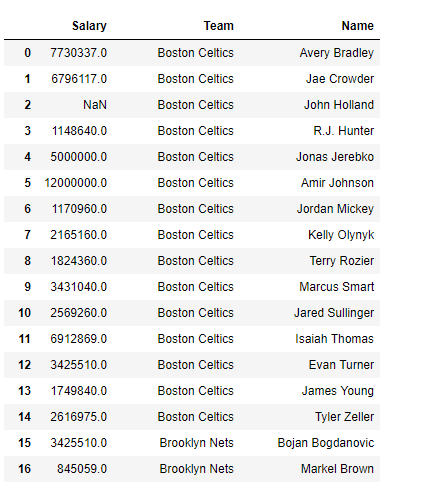# Python | Pandas dataframe.get()

• Last Updated : 19 Nov, 2018

Python is a great language for doing data analysis, primarily because of the fantastic ecosystem of data-centric python packages. Pandas is one of those packages and makes importing and analyzing data much easier.

Pandas` dataframe.get()` function is used to get item from object for given key. The key could be one or more than one dataframe column. It returns default value if not found.

Attention geek! Strengthen your foundations with the Python Programming Foundation Course and learn the basics.

To begin with, your interview preparations Enhance your Data Structures concepts with the Python DS Course. And to begin with your Machine Learning Journey, join the Machine Learning - Basic Level Course

Syntax: DataFrame.get(key, default=None)

Parameters :
key : object

Returns : value : type of items contained in object

Example #1: Use `get()` function to extract a column out of the dataframe

 `# importing pandas as pd``import` `pandas as pd`` ` `# Creating the dataframe ``df ``=` `pd.read_csv(``"nba.csv"``)`` ` `# Print the dataframe``df`

Output :Now apply the `get()` function. We are going to extract the “Salary” column from the dataframe.

 `# applying get() function ``df.get(``"Salary"``)`

Output :Notice, the output is not a dataframe but a pandas series object.

Example #2: Use `get()` function to extract multiple columns at a time in random order

 `# importing pandas as pd``import` `pandas as pd`` ` `# Creating the dataframe ``df ``=` `pd.read_csv(``"nba.csv"``)`` ` `df.get([``"Salary"``, ``"Team"``, ``"Name"``])`

Output :A dataframe is returned as output. The ordering of the columns is not according to the actual dataframe but it follows the ordering that we provided to it in the function input.

My Personal Notes arrow_drop_up